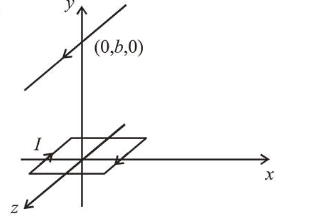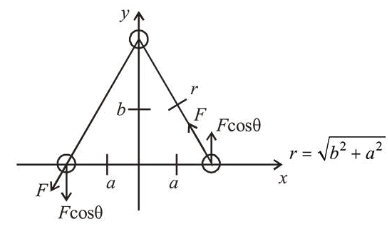# A square loop of side $2 a$ and carrying current I is kept is x z plane with its centre at origin.Question:

A square loop of side $2 a$ and carrying current $\mathrm{I}$ is kept is $x z$ plane with its centre at origin. A long wire carrying the same current $\mathrm{I}$ is placed parallel to $z$-axis and passing through point $(0, b, 0),(b>>a)$. The magnitude of torque on the loop about z-axis will be :

1. (1) $\frac{2 \mu_{0} \mathrm{I}^{2} a^{2}}{\pi b}$

2. (2) $\frac{2 \mu_{0} I^{2} a^{2} b}{\pi\left(a^{2}+b^{2}\right)}$

3. (3) $\frac{\mu_{0} I^{2} a^{2} b}{2 \pi\left(a^{2}+b^{2}\right)}$

4. (4) $\frac{\mu_{0} \mathrm{I}^{2} a^{2}}{2 \pi b}$

Correct Option: , 2

Solution:

(2)Force, $F=B I 2 a=\frac{\mu_{0} I}{2 \pi r} I \times 2 a$

Force, $F=\frac{\mu_{0} I^{2} a}{\pi \sqrt{b^{2}+a^{2}}}$

Torque, $\tau=F_{1} \times$ Perpendicular distance $=F \cos \theta \times 2 a$

$=\frac{\mu_{0} I^{2} a}{\pi \sqrt{b^{2}+a^{2}}} \times \frac{b}{\sqrt{b^{2}+a^{2}}} \times 2 a$

$\Rightarrow \tau=\frac{2 \mu_{0} I^{2} a^{2} b}{\pi\left(a^{2}+b^{2}\right)}$

If $b \gg a$ then $\tau=\frac{2 \mu_{0} I^{2} a^{2}}{\pi b}$